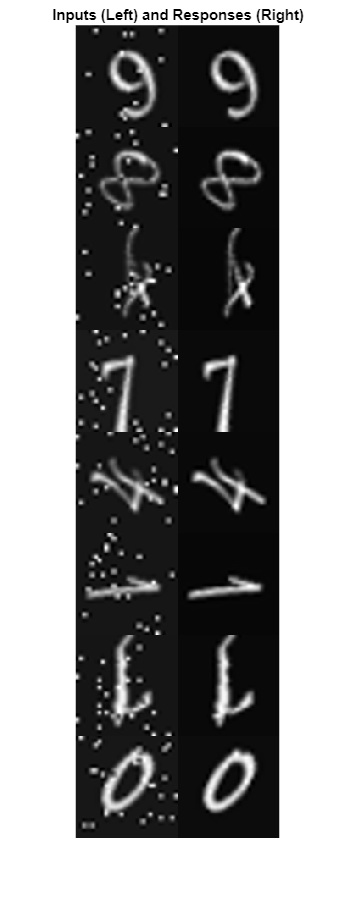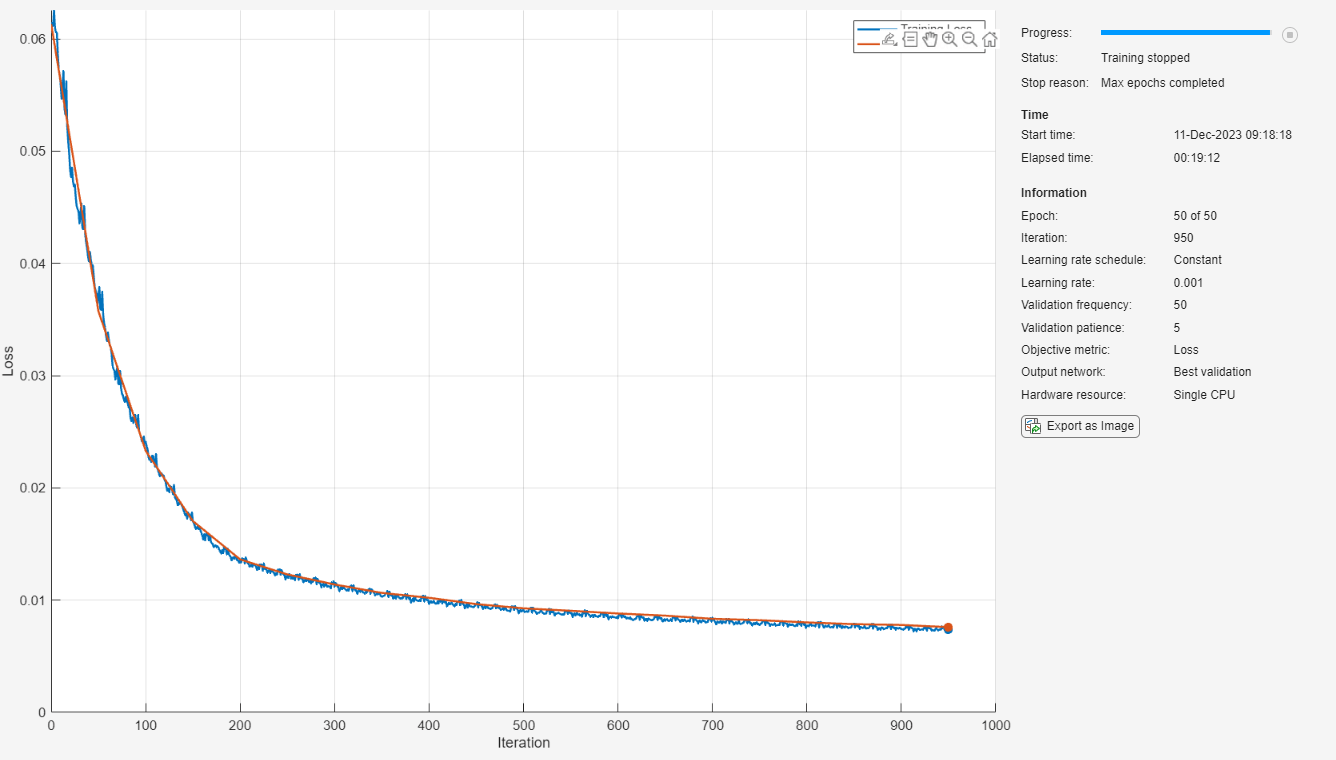# Prepare Datastore for Image-to-Image Regression

This example shows how to prepare a datastore for training an image-to-image regression network using the `transform` and `combine` functions of `ImageDatastore`.

This example shows how to preprocess data using a pipeline suitable for training a denoising network. This example then uses the preprocessed noise data to train a simple convolutional autoencoder network to remove image noise.

### Prepare Data Using Preprocessing Pipeline

This example uses a salt and pepper noise model in which a fraction of input image pixels are set to either `0` or `1` (black and white, respectively). Noisy images act as the network input. Pristine images act as the expected network response. The network learns to detect and remove the salt and pepper noise.

Load the pristine images in the digit data set as an `imageDatastore`. The datastore contains 10,000 synthetic images of digits from 0 to 9. The images are generated by applying random transformations to digit images created with different fonts. Each digit image is 28-by-28 pixels. The datastore contains an equal number of images per category.

```digitDatasetPath = fullfile(matlabroot,"toolbox","nnet", ... "nndemos","nndatasets","DigitDataset"); imds = imageDatastore(digitDatasetPath, ... IncludeSubfolders=true,LabelSource="foldernames");```

Specify a large read size to minimize the cost of file I/O.

`imds.ReadSize = 500;`

Use the `shuffle` function to shuffle the digit data prior to training.

`imds = shuffle(imds);`

Use the `splitEachLabel` function to divide `imds` into three image datastores containing pristine images for training, validation, and testing.

`[imdsTrain,imdsVal,imdsTest] = splitEachLabel(imds,0.95,0.025);`

Use the `transform` function to create noisy versions of each input image, which will serve as the network input. The `transform` function reads data from an underlying datastore and processes the data using the operations defined in the helper function `addNoise` (defined at the end of this example). The output of the `transform` function is a `TransformedDatastore`.

```dsTrainNoisy = transform(imdsTrain,@addNoise); dsValNoisy = transform(imdsVal,@addNoise); dsTestNoisy = transform(imdsTest,@addNoise);```

Use the `combine` function to combine the noisy images and pristine images into a single datastore that feeds data to `trainNetwork`. This combined datastore reads batches of data into a two-column cell array as expected by `trainNetwork`. The output of the `combine` function is a `CombinedDatastore`.

```dsTrain = combine(dsTrainNoisy,imdsTrain); dsVal = combine(dsValNoisy,imdsVal); dsTest = combine(dsTestNoisy,imdsTest);```

Use the `transform` function to perform additional preprocessing operations that are common to both the input and response datastores. The `commonPreprocessing` helper function (defined at the end of this example) resizes input and response images to 32-by-32 pixels to match the input size of the network, and normalizes the data in each image to the range [0, 1].

```dsTrain = transform(dsTrain,@commonPreprocessing); dsVal = transform(dsVal,@commonPreprocessing); dsTest = transform(dsTest,@commonPreprocessing);```

Finally, use the `transform` function to add randomized augmentation to the training set. The `augmentImages` helper function (defined at the end of this example) applies randomized 90 degree rotations to the data. Identical rotations are applied to the network input and corresponding expected responses.

`dsTrain = transform(dsTrain,@augmentImages);`

Augmentation reduces overfitting and adds robustness to the presence of rotations in the trained network. Randomized augmentation is not needed for the validation or test data sets.

### Preview Preprocessed Data

Since there are several preprocessing operations necessary to prepare the training data, preview the preprocessed data to confirm it looks correct prior to training. Use the `preview` function to preview the data.

Visualize examples of paired noisy and pristine images using the `montage` (Image Processing Toolbox) function. The training data looks correct. Salt and pepper noise appears in the input images in the left column. Other than the addition of noise, the input image and response image are the same. Randomized 90 degree rotation is applied to both input and response images in the same way.

```exampleData = preview(dsTrain); inputs = exampleData(:,1); responses = exampleData(:,2); minibatch = cat(2,inputs,responses); montage(minibatch',Size=[8 2]) title("Inputs (Left) and Responses (Right)")```### Define Convolutional Autoencoder Network

Convolutional autoencoders are a common architecture for denoising images. Convolutional autoencoders consist of two stages: an encoder and a decoder. The encoder compresses the original input image into a latent representation that is smaller in width and height, but deeper in the sense that there are many feature maps per spatial location than the original input image. The compressed latent representation loses some amount of spatial resolution in its ability to recover high frequency features in the original image, but it also learns to not include noisy artifacts in the encoding of the original image. The decoder repeatedly upsamples the encoded signal to move it back to its original width, height, and number of channels. Since the encoder removes noise, the decoded final image has fewer noise artifacts.

This example defines the convolutional autoencoder network using layers from Deep Learning Toolbox™, including:

Create the image input layer. To simplify the padding concerns related to downsampling and upsampling by factors of two, choose a 32-by-32 input size because 32 is cleanly divisible by 2, 4, and 8.

`imageLayer = imageInputLayer([32,32,1]);`

Create the encoding layers. Downsampling in the encoder is achieved by max pooling with a pool size of 2 and a stride of 2.

```encodingLayers = [ ... convolution2dLayer(3,8,Padding="same"), ... reluLayer, ... maxPooling2dLayer(2,Padding="same",Stride=2), ... convolution2dLayer(3,16,Padding="same"), ... reluLayer, ... maxPooling2dLayer(2,Padding="same",Stride=2), ... convolution2dLayer(3,32,Padding="same"), ... reluLayer, ... maxPooling2dLayer(2,Padding="same",Stride=2)];```

Create the decoding layers. The decoder upsamples the encoded signal using a transposed convolution layer with a stride of 2, which upsamples by a factor of 2. The network uses a `clippedReluLayer` as the final activation layer to force outputs to be in the range [0, 1].

```decodingLayers = [ ... transposedConv2dLayer(2,32,Stride=2), ... reluLayer, ... transposedConv2dLayer(2,16,Stride=2), ... reluLayer, ... transposedConv2dLayer(2,8,Stride=2), ... reluLayer, ... convolution2dLayer(1,1,Padding="same"), ... clippedReluLayer(1.0), ... regressionLayer]; ```

Concatenate the image input layer, the encoding layers, and the decoding layers to form the convolutional autoencoder network architecture.

`layers = [imageLayer,encodingLayers,decodingLayers];`

### Define Training Options

Train the network using Adam optimization. Specify the hyperparameter settings by using the `trainingOptions` function. Train for 50 epochs.

```options = trainingOptions("adam", ... MaxEpochs=50, ... MiniBatchSize=imds.ReadSize, ... ValidationData=dsVal, ... ValidationPatience=5, ... Plots="training-progress", ... OutputNetwork="best-validation-loss", ... Verbose=false);```

### Train the Network

Now that the data source and training options are configured, train the convolutional autoencoder network using the `trainNetwork` function.

Train on a GPU if one is available. Using a GPU requires Parallel Computing Toolbox™ and a CUDA® enabled NVIDIA® GPU. For more information, see GPU Computing Requirements (Parallel Computing Toolbox). Training takes about 15 minutes on an NVIDIA Titan XP.

```net = trainNetwork(dsTrain,layers,options); modelDateTime = string(datetime("now",Format="yyyy-MM-dd-HH-mm-ss")); save("trainedImageToImageRegressionNet-"+modelDateTime+".mat","net"); ```

### Evaluate the Performance of the Denoising Network

Obtain output images from the test set by using the `predict` function.

`ypred = predict(net,dsTest);`

Obtain pairs of noisy and pristine images from the test set using the `preview` function.

`testBatch = preview(dsTest);`

Visualize a sample input image and the associated predicted output from the network to get a sense of how well denoising is working. As expected, the output image from the network has removed most of the noise artifacts from the input image. The denoised image is slightly blurry as a result of the encoding and decoding process.

```idx = 1; y = ypred(:,:,:,idx); x = testBatch{idx,1}; ref = testBatch{idx,2}; montage({x,y})```Assess the performance of the network by analyzing the peak signal-to-noise ratio (PSNR).

`psnrNoisy = psnr(x,ref)`
```psnrNoisy = single 19.6457 ```
`psnrDenoised = psnr(y,ref)`
```psnrDenoised = single 20.6994 ```

The PSNR of the output image is higher than the noisy input image, as expected.

### Supporting Functions

The `addNoise` helper function adds salt and pepper noise to images by using the `imnoise` (Image Processing Toolbox) function. The `addNoise` function requires the format of the input data to be a cell array of image data, which matches the format of data returned by the `read` function of `ImageDatastore`.

```function dataOut = addNoise(data) dataOut = data; for idx = 1:size(data,1) dataOut{idx} = imnoise(data{idx},"salt & pepper"); end end```

The `commonPreprocessing` helper function defines the preprocessing that is common to the training, validation, and test sets. The helper function performs these preprocessing steps.

1. Convert the image data to data type `single`.

2. Resize image data to match the size of the input layer by using the `imresize` function.

3. Normalize data to the range [0, 1] by using the `rescale` function.

The helper function requires the format of the input data to be a two-column cell array of image data, which matches the format of data returned by the `read` function of `CombinedDatastore`.

```function dataOut = commonPreprocessing(data) dataOut = cell(size(data)); for col = 1:size(data,2) for idx = 1:size(data,1) temp = single(data{idx,col}); temp = imresize(temp,[32,32]); temp = rescale(temp); dataOut{idx,col} = temp; end end end```

The `augmentImages` helper function adds randomized 90 degree rotations to the data by using the `rot90` function. Identical rotations are applied to the network input and corresponding expected responses. The function requires the format of the input data to be a two-column cell array of image data, which matches the format of data returned by the `read` function of `CombinedDatastore`.

```function dataOut = augmentImages(data) dataOut = cell(size(data)); for idx = 1:size(data,1) rot90Val = randi(4,1,1)-1; dataOut(idx,:) = {rot90(data{idx,1},rot90Val), ... rot90(data{idx,2},rot90Val)}; end end```